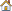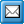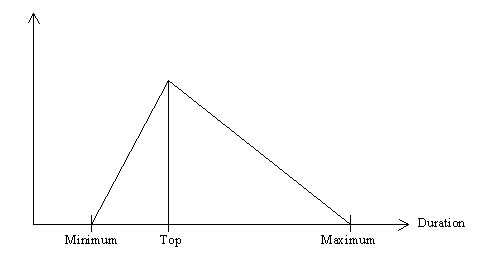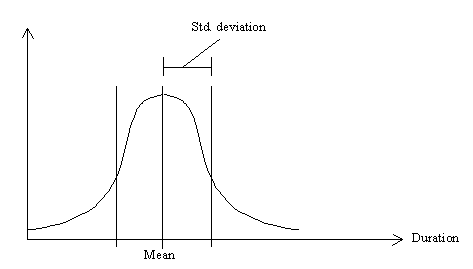﻿ Distributions
QPR Knowledge Base 2017.1

DistributionsModeling Client User's Guide > Simulation > Preparing a Simulation:

DistributionsDistributionsThe processing times and input frequencies have four different distributions to select from: constant, triangular, normal and user defined. The constant distribution is the simplest of the four: the duration of the activation is always the same. The triangular distribution generates a random number so that the number is between the minimum and maximum values with the stated peak or top value.Triangular distribution

The standard distribution is calculated from the mean value and standard deviation values.Standard distribution

The user-defined distribution consists of a list of values that are used in the given order, going back to the first value after all values have been used.